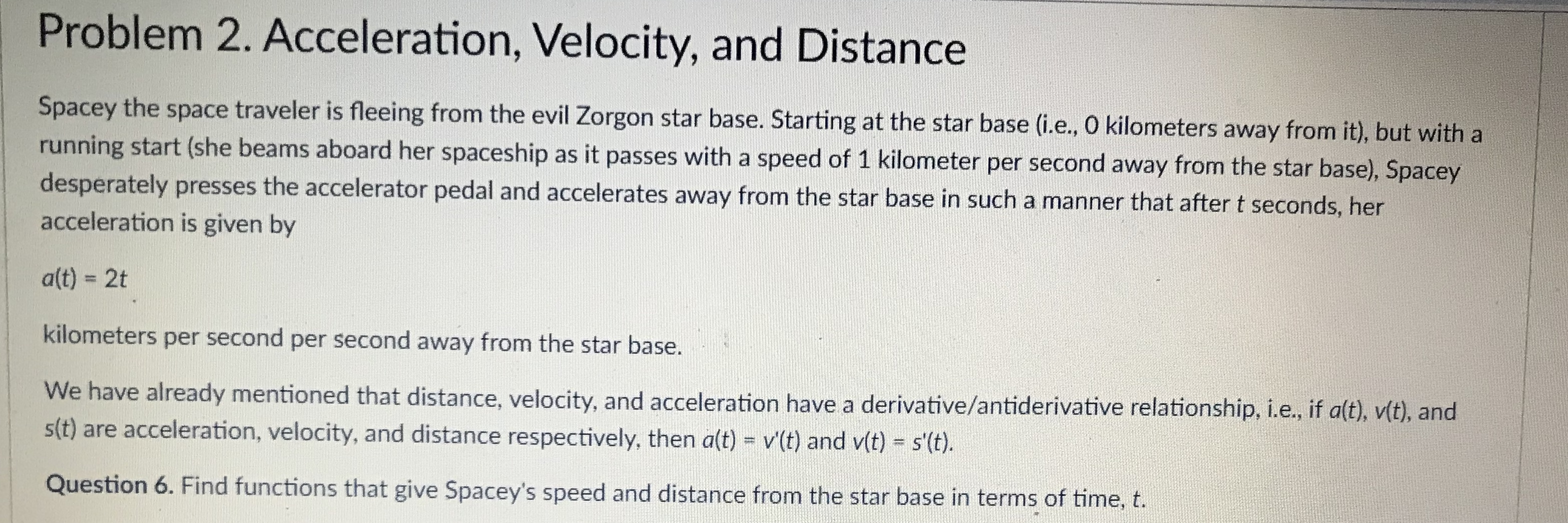# Problem 2. Acceleration, Velocity, and DistanceSpacey the space traveler is fleeing from the evil Zorgon star base. Starting at the star base (i.e., O kilometers away from it), but with arunning start (she beams aboard hdesperately presses the accelerator pedal and accelerates away from the star base in such a manner that after t seconds, heracceleration is given byer spaceship as it passes with a speed of 1 kilometer per second away from the star base), Spaceyalt) - 2tkilometers per second per second away from the star base.We have already mentioned that distance, velocity, and accelerationst) are acceleration, velocity, and distance respectively, then aft) - vt) and v(t)-st.Question 6. Find functions that give Spacey's speed and distance from the star base in terms of time, t.have a derivative/antiderivative relationship, i.e, if alt), vit), and

Question
14 views

How do you solve number 6?help_outlineImage TranscriptioncloseProblem 2. Acceleration, Velocity, and Distance Spacey the space traveler is fleeing from the evil Zorgon star base. Starting at the star base (i.e., O kilometers away from it), but with a running start (she beams aboard h desperately presses the accelerator pedal and accelerates away from the star base in such a manner that after t seconds, her acceleration is given by er spaceship as it passes with a speed of 1 kilometer per second away from the star base), Spacey alt) - 2t kilometers per second per second away from the star base. We have already mentioned that distance, velocity, and acceleration st) are acceleration, velocity, and distance respectively, then aft) - vt) and v(t)-st. Question 6. Find functions that give Spacey's speed and distance from the star base in terms of time, t. have a derivative/antiderivative relationship, i.e, if alt), vit), and fullscreen
check_circle

Step 1

Given:

The Spacey's accelaration with respect to time t is a(t) = 2t.

The Spacey's speed and distances is represented as v(t) and s(t), respecively.

The rate of change of speed with respect to time is the accelaration. That is, a(t) = v'(t).

Similarly, the rate of change of distance with respect to time is the speed. That is, v(t) = s'(t).

Step 2
Step 3

Calculation:

The Spacey\'s speed is computed as follows.

Since a(t) = v\'(t), substitute a(t) = 2t in it.

2t = v\'(t)

Inte...

### Want to see the full answer?

See Solution

#### Want to see this answer and more?

Solutions are written by subject experts who are available 24/7. Questions are typically answered within 1 hour.*

See Solution
*Response times may vary by subject and question.
Tagged in

### Other